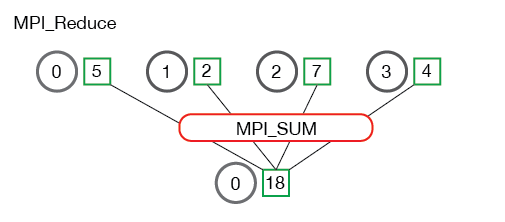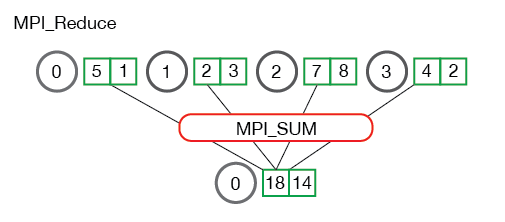# MPI Reduce and Allreduce

Author: Wes Kendall

Note - 本教程的所有代码都在 GitHub 上。本教程的代码位于 tutorials/mpi-reduce-and-allreduce/code 下。

## MPI_Reduce

`MPI_Gather` 类似，`MPI_Reduce` 在每个进程上获取一个输入元素数组，并将输出元素数组返回给根进程。 输出元素包含减少的结果。 `MPI_Reduce` 的原型如下所示：

``````MPI_Reduce(
void* send_data,
void* recv_data,
int count,
MPI_Datatype datatype,
MPI_Op op,
int root,
MPI_Comm communicator)
``````

`send_data` 参数是每个进程都希望归约的 `datatype` 类型元素的数组。 `recv_data` 仅与具有 `root` 秩的进程相关。 `recv_data` 数组包含归约的结果，大小为`sizeof（datatype）* count``op` 参数是您希望应用于数据的操作。 MPI 包含一组可以使用的常见归约运算。 尽管可以定义自定义归约操作，但这超出了本教程的范围。 MPI 定义的归约操作包括：

• `MPI_MAX` - 返回最大元素。
• `MPI_MIN` - 返回最小元素。
• `MPI_SUM` - 对元素求和。
• `MPI_PROD` - 将所有元素相乘。
• `MPI_LAND` - 对元素执行逻辑运算。
• `MPI_LOR` - 对元素执行逻辑运算。
• `MPI_BAND` - 对元素的各个位按位执行。
• `MPI_BOR` - 对元素的位执行按位运算。
• `MPI_MAXLOC` - 返回最大值和所在的进程的秩。
• `MPI_MINLOC` - 返回最小值和所在的进程的秩。## 使用 MPI_Reduce 计算均值

``````float *rand_nums = NULL;
rand_nums = create_rand_nums(num_elements_per_proc);

// Sum the numbers locally
float local_sum = 0;
int i;
for (i = 0; i < num_elements_per_proc; i++) {
local_sum += rand_nums[i];
}

// Print the random numbers on each process
printf("Local sum for process %d - %f, avg = %f\n",
world_rank, local_sum, local_sum / num_elements_per_proc);

// Reduce all of the local sums into the global sum
float global_sum;
MPI_Reduce(&local_sum, &global_sum, 1, MPI_FLOAT, MPI_SUM, 0,
MPI_COMM_WORLD);

// Print the result
if (world_rank == 0) {
printf("Total sum = %f, avg = %f\n", global_sum,
global_sum / (world_size * num_elements_per_proc));
}
``````

``````>>> cd tutorials
>>> ./run.py reduce_avg
mpirun -n 4  ./reduce_avg 100
Local sum for process 0 - 51.385098, avg = 0.513851
Local sum for process 1 - 51.842468, avg = 0.518425
Local sum for process 2 - 49.684948, avg = 0.496849
Local sum for process 3 - 47.527420, avg = 0.475274
Total sum = 200.439941, avg = 0.501100
``````

## MPI_Allreduce

``````MPI_Allreduce(
void* send_data,
void* recv_data,
int count,
MPI_Datatype datatype,
MPI_Op op,
MPI_Comm communicator)
``````

`MPI_Allreduce` 等效于先执行 `MPI_Reduce`，然后执行 `MPI_Bcast`。 很简单，对吧？

## 使用 MPI_Allreduce 计算标准差

``````rand_nums = create_rand_nums(num_elements_per_proc);

// Sum the numbers locally
float local_sum = 0;
int i;
for (i = 0; i < num_elements_per_proc; i++) {
local_sum += rand_nums[i];
}

// Reduce all of the local sums into the global sum in order to
// calculate the mean
float global_sum;
MPI_Allreduce(&local_sum, &global_sum, 1, MPI_FLOAT, MPI_SUM,
MPI_COMM_WORLD);
float mean = global_sum / (num_elements_per_proc * world_size);

// Compute the local sum of the squared differences from the mean
float local_sq_diff = 0;
for (i = 0; i < num_elements_per_proc; i++) {
local_sq_diff += (rand_nums[i] - mean) * (rand_nums[i] - mean);
}

// Reduce the global sum of the squared differences to the root
// process and print off the answer
float global_sq_diff;
MPI_Reduce(&local_sq_diff, &global_sq_diff, 1, MPI_FLOAT, MPI_SUM, 0,
MPI_COMM_WORLD);

// The standard deviation is the square root of the mean of the
// squared differences.
if (world_rank == 0) {
float stddev = sqrt(global_sq_diff /
(num_elements_per_proc * world_size));
printf("Mean - %f, Standard deviation = %f\n", mean, stddev);
}
``````

``````>>> ./run.py reduce_stddev
mpirun -n 4  ./reduce_stddev 100
Mean - 0.501100, Standard deviation = 0.301126
``````

## Want to contribute?

This site is hosted entirely on GitHub. This site is no longer being actively contributed to by the original author (Wes Kendall), but it was placed on GitHub in the hopes that others would write high-quality MPI tutorials. Click here for more information about how you can contribute.